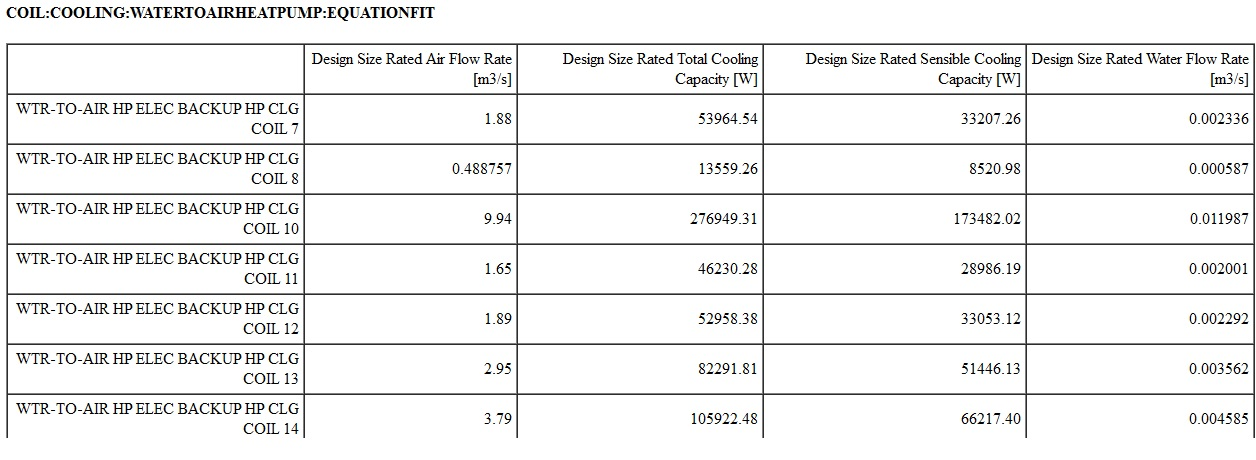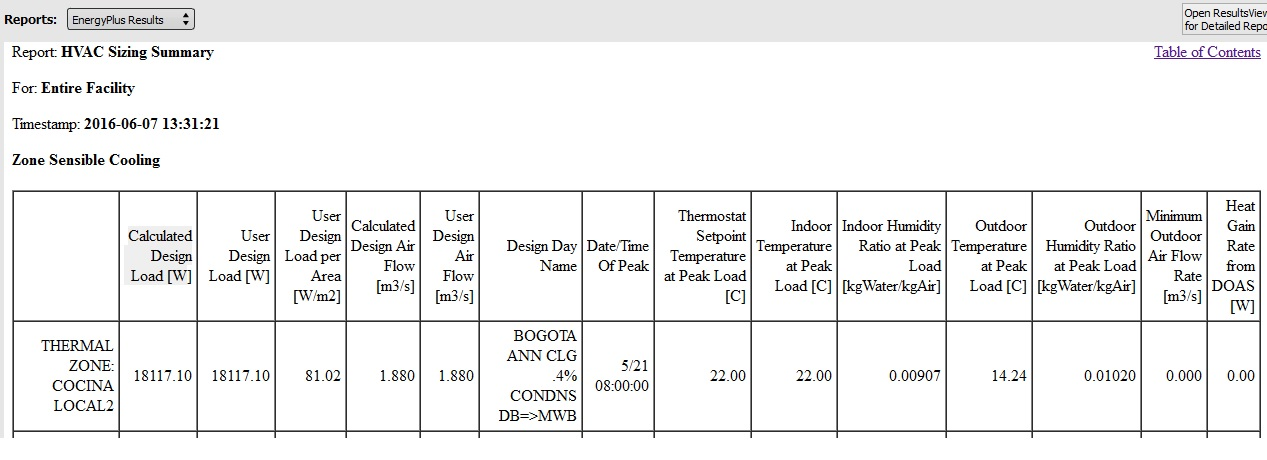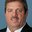Question-and-Answer Resource for the Building Energy Modeling Community
Get s tarted with the Help page

# design size rated cooling vs calculated design loadHi,

In a project, a thermal zone with a doa and a default water to air heat pump, cooling thermostat 22°C, the maximum outdoor temp is 21°C, and density correction fctor is 0.731, the resultant are:

Design Size Rated Total Cooling Capacity 53964 W(the first)

CALCULATED DESIGN LOAD 18,117 WIf the there is no heat gain from DOA, why is the difference between results?

There is no safety factor in the project.

How can i attach a project?

edit retag close merge delete

Please tag with software you are using. Also, please try to avoid creating new tags. Nobody is subscribed to the tag 'vs'. Thanks.

OPEN STUDIO 1.10.0, because this version allow me to select air cooled chillers with evaporative condenser and the latest version doesn't allow this.

Sort by » oldest newest most votedYou could try turning off the DOA and try this again. I have found that when DOA is used, the entering humidity ratio used for sizing the cooling coil is higher than it should be since the "zone" does have outdoor air supplied to the space even thought it is not through the cooling coil. Note the indoor humidity ratio shown above = 0.009 when the outdoor humidity ratio = 0.01. A zone with DOA should have a lower humidity ratio than shown here. For example, 22C DBT/50% RH = 0.008. The higher zone humidity ratio causes a larger cooling coil size.

The other input that affects coil size is the total cooling capacity coefficients which are used during sizing. Coil:Cooling:WaterToAirHeatPump:EquationFit, N6, \field Total Cooling Capacity Coefficient 1 N7, \field Total Cooling Capacity Coefficient 2 N8, \field Total Cooling Capacity Coefficient 3 N9, \field Total Cooling Capacity Coefficient 4 N10, \field Total Cooling Capacity Coefficient 5

Calculate the correction factor yourself to see if these inputs have an impact on coil sizing:

ratioTWB = ( MixWetBulb + 273.15 ) / 283.15;
ratioTS = ( ( ( 85.0 - 32.0 ) / 1.8 ) + 273.15 ) / 283.15;
TotCapTempModFac = TotalCapCoeff1 + ( ratioTWB * TotalCapCoeff2 ) + ( ratioTS * TotalCapCoeff3 ) + ( 1.0 * TotalCapCoeff4 ) + ( 1.0 * TotalCapCoeff5 );
CoolCapAtPeak = rhoair * VolFlowRate * ( MixEnth - SupEnth );
RatedCapCoolTotalDes = CoolCapAtPeak / TotCapTempModFac;

more

Is there a way to see the psycrometric calculation for the resultant? In the other hand, where can i fin this coefficient for water to air heath pump or any reference? the manufacturer catalog only show performance data, i tried to use the excel coefficient calculation included in energyplus and the resultant was out of range.

I Turned of the DOA and the result was the same, because the outdoor condition is better than indoor condition, notice the lower outdoor drybulb temp at peak condition.

The coefficients are created from manufacturers performance data. These are a curve fit of the performance data over a range of operating conditions.

See this doc for an example of how to do this in excel for VRF systems on page 10 and 11.

All you would need to do is change the equation to match the TotCapTempModFac equation above. And each column represents the term in this equation (I see 4 above since the a0 term [or TotalCapCoeff1] isn't used).

ratioTDB = ( ( LoadDBT + 273.15 ) / 283.15 );
ratioTWB = ( ( LoadWBT + 273.15  ) / Tref );
ratioTS = ( ( SourceInletTemp + 273.15  ) / Tref );


The five columns in your spreadsheet would represent these variables. You would regress the last 4 versus the first to get your 5 coefficients.

    (QLoad / TotalCapRated),  ratioTWB,  ratioTS,  ratioVL,  ratioVS